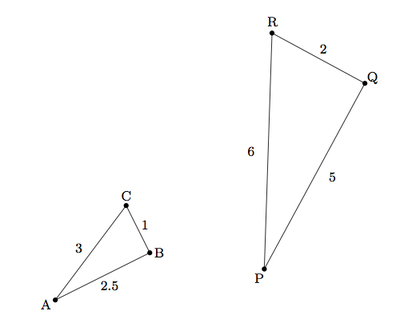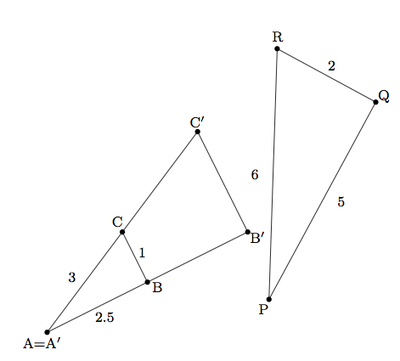# Similar Triangles

Alignments to Content Standards: G-SRT.A.2

Alicia has two triangles $ABC$ and $PQR$ whose corresponding sides are proportional as pictured below:Alicia says:

I wonder if they are similar because I don't have any information about the angles?

What is the answer to Alicia's question? Explain.

## IM Commentary

The goal of this task is to examine similarity of triangles from the point of view of proportional sides, showing that this is sufficient to conclude that two triangles are similar. There are two natural approaches:

• Use a dilation and then apply the SSS criterion for triangle congruence
• Use a dilation and then a series of rigid motions (in essence showing that the SSS criterion holds for these particular triangles).

Only the first of these is presented here. For the second method, consult http://www.illustrativemathematics.org/illustrations/110. While the dilation does not have to be applied first, it is natural to do so because we are not given any information about the angles and so the most we can do with what is given is align two vertices of the triangles. If students are not already familiar with the SSS congruence criterion for triangles, then they should demonstrate the congruence between $\triangle A^\prime B^\prime C^\prime$ and $\triangle PQR$ as outlined in the solution.

It is interesting to note that for two triangles, either congruent angles or proportional sides is sufficient to conclude that they are similar. In other words, three congruent angles implies three proportional sides and vice versa.

## Solution

In order to determine whether $\triangle ABC$ and $\triangle PQR$ are similar, we need to find a sequence of dilations and rigid motions which will map $\triangle ABC$ to $\triangle PQR$. We can see that the sides of $\triangle PQR$ are each twice as long as the corresponding sides of $\triangle ABC$ so we can begin by dilating $\triangle ABC$ with center of dilation $A$, by a scale factor of 2, giving $\triangle A^\prime B^\prime C^\prime$ shown below:Since $\triangle A^\prime B^\prime C^\prime$ has the same side lengths as $\triangle PQR$ (as we see by doubling the side lengths of $\triangle ABC$) they are congruent by SSS. So we can move $\triangle A^\prime B^\prime C^\prime$ to $\triangle PQR$ with translations, rotations, and reflections: in fact, we can translate $A^\prime$ to $P$ and then a rotation will place this new triangle on top of $\triangle PQR$. This means that with a dilation followed by a translation and a rotation, $\triangle ABC$ maps to $\triangle PQR$ and so these two triangles are similar.